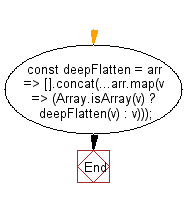# JavaScript: Deep flatten an array

## JavaScript fundamental (ES6 Syntax): Exercise-248 with Solution

Write a JavaScript program to deep flatten an array.

• Use recursion.
• Use Array.prototype.concat() with an empty array ([]) and the spread operator (...) to flatten an array.
• Recursively flatten each element that is an array.

Sample Solution:

JavaScript Code:

``````//#Source https://bit.ly/2neWfJ2
const deepFlatten = arr => [].concat(...arr.map(v => (Array.isArray(v) ? deepFlatten(v) : v)));
console.log(deepFlatten([1, , [, 4], 5]));
```
```

Sample Output:

```[1,2,3,4,5]
```

Flowchart:Live Demo:

See the Pen javascript-basic-exercise-248-1 by w3resource (@w3resource) on CodePen.

Improve this sample solution and post your code through Disqus

What is the difficulty level of this exercise?

Test your Programming skills with w3resource's quiz.

﻿